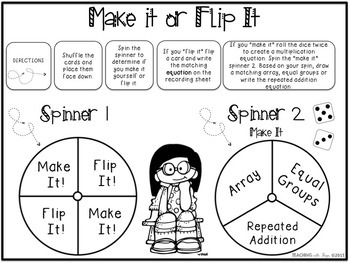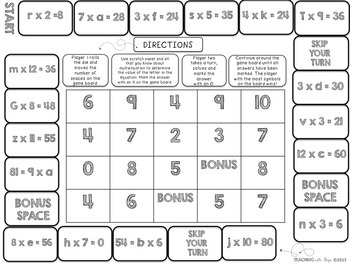# Multiplication Centers Multiplication Games

Rated 4.94 out of 5, based on 181 reviews
181 Ratings;
3rd - 5th
Subjects
Resource Type
Standards
Formats Included
• PDF
Pages
40 pages
Report this resource to TPT

### Description

I know your students will love these centers as much as mine have!
I designed these centers to be engaging and purposeful. If students are engaged in meaningful practice I am free to run small math groups that maximize student learning.

In this download you will find 10 centers. These centers will help students practice their beginning multiplication skills including; arrays, equal groups, skip counting, repeated addition, missing factors, equations with three factors, comparing products, and more.

You might also be interested in:

Multiplication Centers: Advanced Skills for math center practice with multiplication properties and more!

Multiplication Centers: The Bundle

Multiplication Exit Slips

Each center has student friendly directions right on the game board or recording sheet. The materials for each center are minimal. Students may need a paperclip, pencil, dice, any item that will serve as a game piece, and cards (included).

Centers:

Roll A Product: Use dice to create an equation. Using the recording sheet and example. Show the equation as an array, equal groups, repeated addition and finally solve for the product.

Times Tac Toe and Challenge Times Tac Toe: In this partner game students strategically move game pieces from factor to factor to create a product. They mark their answer on the game board trying to beat their partner to creating a vertical, horizontal or diagonal row.

Multiplication Solve & Sort: Students shuffle cards, choose a card and solve it using the recording sheet then sort the products on the sorting mat.

Multiplication Roll n’ Spin: Students use a die to roll one factor and the spinner on the game board to spin the other. They solve to find the product and then determine whether the answer is odd or even.

Three Factors: This game lays out a simple way for students to practice multiplying three factors. Students roll a die to create their equation and then the game board leads them step by step to solving to find the product.

Make it or Flip It: Students spin the spinner to determine whether they make the equation themselves or if they get to “Flip It”, flip a card instead. If they flip a card they follow the directions on the card. But, if they make it themselves they have to spin the second spinner to determine whether they make an array, equal groups or repeated addition.

Missing Factor Race: With a partner, students race around the game board solving equations with missing factors. Who will have the most symbols on the game board when the race is finished?

Multiplication War: This is played like traditional war, but with multiplication cards. This is a simple game, but it is always amazing to me how many kids have never played this game. The multiplication cards and directions are of course, included!

Fact Family Flip: Students flip cards containing fact families. They record the multiplication and division equations on the recording sheet for each fact family.

Solve n’ Compare: Using the spinner, students create two different equations. They solve each equation and then compare the answers using greater than, less than, and =

Check out the preview for a closer look!
Total Pages
40 pages
N/A
Teaching Duration
N/A
Report this resource to TPT
Reported resources will be reviewed by our team. Report this resource to let us know if this resource violates TPT’s content guidelines.

### Standards

to see state-specific standards (only available in the US).
Interpret products of whole numbers, e.g., interpret 5 × 7 as the total number of objects in 5 groups of 7 objects each. For example, describe a context in which a total number of objects can be expressed as 5 × 7.
Interpret whole-number quotients of whole numbers, e.g., interpret 56 ÷ 8 as the number of objects in each share when 56 objects are partitioned equally into 8 shares, or as a number of shares when 56 objects are partitioned into equal shares of 8 objects each. For example, describe a context in which a number of shares or a number of groups can be expressed as 56 ÷ 8.
Use multiplication and division within 100 to solve word problems in situations involving equal groups, arrays, and measurement quantities, e.g., by using drawings and equations with a symbol for the unknown number to represent the problem.
Determine the unknown whole number in a multiplication or division equation relating three whole numbers. For example, determine the unknown number that makes the equation true in each of the equations 8 × ? = 48, 5 = __ ÷ 3, 6 × 6 = ?.
Apply properties of operations as strategies to multiply and divide. Examples: If 6 × 4 = 24 is known, then 4 × 6 = 24 is also known. (Commutative property of multiplication.) 3 × 5 × 2 can be found by 3 × 5 = 15, then 15 × 2 = 30, or by 5 × 2 = 10, then 3 × 10 = 30. (Associative property of multiplication.) Knowing that 8 × 5 = 40 and 8 × 2 = 16, one can find 8 × 7 as 8 × (5 + 2) = (8 × 5) + (8 × 2) = 40 + 16 = 56. (Distributive property.)